# MP Board Class 7th Maths Solutions Chapter 3 Data Handling Ex 3.3

## MP Board Class 7th Maths Solutions Chapter 3 Data Handling Ex 3.3

Question 1.
Use the given bar graph to answer the following questions.
(a) Which is the most popular pet?
(b) How many students have dog as a pet?Solution:
(a) Since, the bar representing number of students for cats is the tallest, so cat is the most popular pet.
(b) The number of students having dog as a pet is 8.Question 2.
Read the given bar graph which shows the number of books sold by a bookstore during five consecutive years and answer the following questions: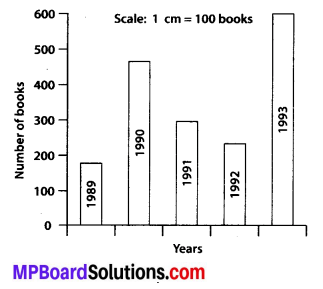(i) About how many books were sold in 1989? 1990? 1992?
(ii) In which year were about 475 books sold? About 225 books sold?
(iii) In which years were fewer than 250 books sold?
(iv) Can you explain how you would estimate the number of books sold in 1989?
Solution:
(i) In 1989, 175 books were sold. In 1990, 475 books were sold. In 1992, 225 books were sold.
(ii) From the graph, it can be concluded that in the year 1990 about 475 books were sold and in the year 1992 about 225 books were sold.
(iii) From the graph, it can be concluded that in the year 1989 and 1992, the number of books sold were less than 250.
(iv) From the graph, it can be concluded that the number of books sold in the year 1989 is about 1 and $$\frac{3}{4}$$ th part of 1 cm.
We know that the scale is taken as 1 cm = 100 books.
∴ Number of books sold in 1989Therefore, about 175 books were sold in the year 1989.Question 3.
Number of children in six different classes are given below. Represent the data on a bar graph.(a) How would you choose a scale?
(i) Which class has the maximum number of children? And the minimum?
(ii) Find the ratio of students of class sixth to the students of class eighth.
Solution: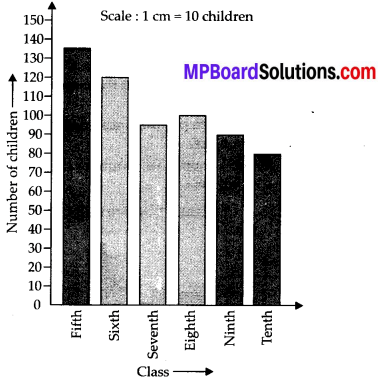(a) We will choose a scale as 1 unit = 10 children because we can represent a more
clear difference between the number of students of class 7th and that of class 9th by this scale.
(b) (i) Since, the bar representing the number of children for class fifth is the tallest. So, there are maximum number of children in class fifth. Similarly, the bar representing the number of children for class tenth is the smallest. So, there are minimum number of children in class tenth.
(ii) The number of students in class sixth is 120 and the number of students in class eighth is 100. Therefore, the ratio of students of class sixth to the students 5.
of class eighth $$=\frac{120}{100}=\frac{6}{5}$$ i.e., 6 : 5Question 4.
The performance of a student in 1st Term and 2nd Term is given. Draw a double bar graph choosing appropriate scale and answer the following: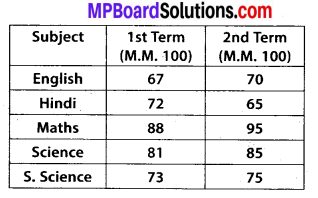(i) In which subject, has the child improved his performance the most?
(ii) In which subject is the improvement the least?
(iii) Has the performance gone down in any subject?
Solution:
A double bar graph for the given data is as follows.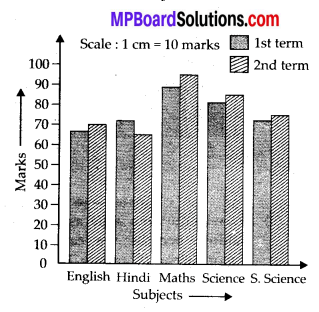(i) There was a maximum increase in the marks obtained in Maths. Therefore, the child has improved his performance the most in Maths.
(ii) From the graph, it can be concluded that the improvement was the least in
S. Science.
(iii) From the graph, it can be observed that the performance in Hindi has gone down.

Question 5.
Consider this data collected from a survey of a colony.(i) Draw a double bar graph choosing an appropriate scale. What do you infer from the bar graph?
(ii) Which sport is most popular?
(iii) Which is more preferred, watching or participating in sports?
Solution:
(i) A double bar graph for the given data is as follows:The double bar graph represents the number of people who like watching and participating in different sports.
(ii) From the bar graph, it can be observed that the bar representing the number of people who like watching and participating in cricket is the tallest among all the bars. Hence, cricket is the most popular sport.
(iii) The bars representing watching sport are longer than the bars representing participating in sport. Hence, watching different types of sports is more preferred than participating in the sports.Question 6.
Take the data giving the minimum and the maximum temperature of various cities given in the table. Plot a double bar graph using the data and answer the following:(i) Which city has the largest difference in the minimum and maximum temperature on the given date?
(ii) Which is the hottest city and which is the coldest city?
(iii) Name two cities where maximum temperature of one was less than the minimum temperature of the other.
(iv) Name the city which has the least difference between its minimum and the maximum temperature.
Solution:
A double bar graph for the given data is constructed as follows.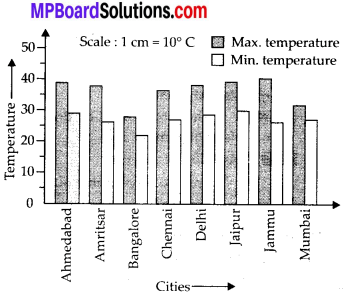(i) From the graph, it can be concluded that Jammu has the largest difference in its minimum and maximum temperature on 20.6.2006.
(ii) From the graph, it can be concluded that Jammu is the hottest city and Bangalore is the coldest city.
(iii) Bangalore and Jaipur, Bangalore and Ahmedabad.
For Bangalore, the maximum temperature was 28°C, while minimum temperature of both cities, Ahmedabad and Jaipur, was 29°C.
(iv) From the graph, it can be concluded that the city which has least difference between its minimum and maximum temperature is Mumbai.